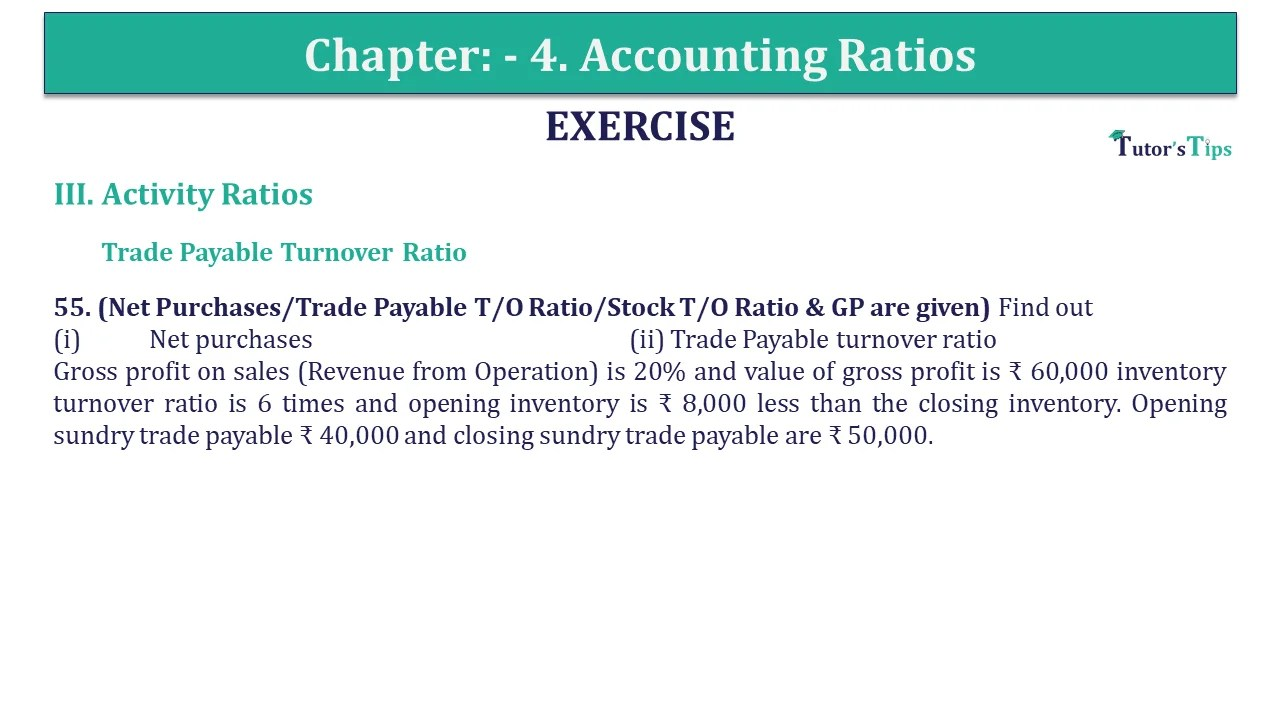# Question 55 Chapter 4 of +2-B – USHA Publication 12 ClassQ-55- CH-4 Book 2 - Usha Pub. +2 Book 2020 - Solution

Question 55 Chapter 4 of +2-B

55. (Net Purchases/Trade Payable T/O Ratio/Stock T/O Ratio & GP are given) Find out
(i) Net purchases (ii) Trade Payable turnover ratio
Gross profit on sales (Revenue from Operation) is 20% and the value of gross profit is ₹ 60,000 inventory turnover ratio is 6 times and the opening inventory is ₹ 8,000 less than the closing inventory. Opening sundry trade payable ₹ 40,000 and closing sundry trade payable is ₹ 50,000.

## The solution of Question 55 Chapter 4 of +2-B: –

 Gross profit = 20% on sales Cost of goods sold = 80% of sales Sales = Cost of goods sold + Profit = ₹ 80+ ₹ 20
 Cost of goods sold = 80 X 60,000 20 = ₹ 2,40,000
 Inventory Turnover Ratio = Cost of goods sold Average inventory 6 = 1,40,000 Average inventory Average Inventory = 2,40,000 6 Average Inventory = ₹ 40,000 Average Inventory = Opening Inventory + Closing Inventory 2 Let assume Closing Inventory = x Opening Inventory = x – ₹ 8,000 Average Inventory = (x – ₹ 8,000) + x 2 ₹ 40,000 = 2x – ₹ 8,000 2 ₹ 40,000 x 2 = 2x – ₹ 8,000 ₹ 80,000 = 2x – ₹ 8,000 ₹ 80,000 + ₹ 8,000 = 2x = ₹ 88,000 2 Closing Inventory = ₹ 44,000 Opening Inventory = Closing Inventory – ₹ 8,000 = ₹ 44,000 – ₹ 8,000 = ₹ 36,000

(a) Computation of net purchases

 Cost of goods sold = Opening Inventory + Purchases – Closing Inventory ₹ 2,40,000 = ₹ 36,000 + Purchases – ₹ 44,000 Purchases = ₹ 2,40,000 + ₹ 8,000 = ₹ 2,48,000

(b) Accounts Payable Turnover Ratio

 = Net Purchases (Credit) Average Accounts Payable Average accounts payable = Opening accounts payable + Closing accounts payable 2 = ₹ 40,000 + ₹ 50,000 2 = ₹ 45,000 = ₹ 2,48,000 Accounts Payable Turnover Ratio ₹ 45,000 = 5.51 times

Also, Check out the solved question of previous Chapters: –